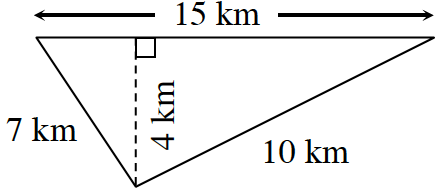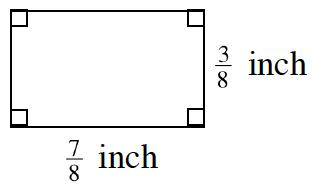### Home > CC1MN > Chapter 8 > Lesson 8.2.2 > Problem8-89

8-89.

Find the perimeter and area of each figure below.

1.• Add all the side lengths together to find the perimeter.

• $7+15+10=?$

• Use the equation for finding the area of a triangle.

• $\text{Area}=\frac{1}{2}\text{ (base)(height)}$

1.• Add all the side lengths together to find the perimeter.
A rectangle has two pairs of sides with equal lengths.

• $\frac{7}{8}+\frac{3}{8}+\frac{7}{8}+\frac{3}{8}=?$

• Use the equation for finding the area of a rectangle.

• Area = (base)(height)

• $\text{Perimeter: }\frac{20}{8}=2.5\text{ inches}$

• $\text{ Area:}\ \frac{21}{64}\text{ in}^{2}$